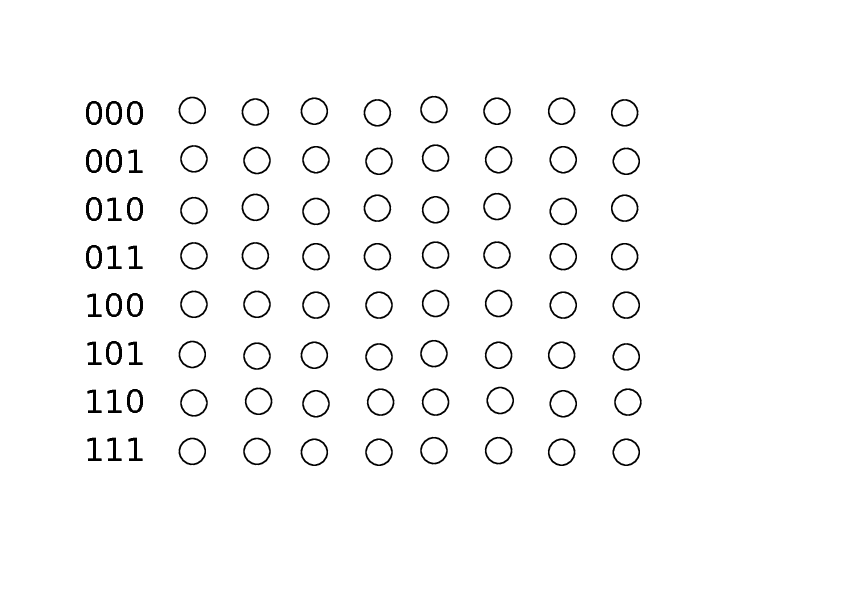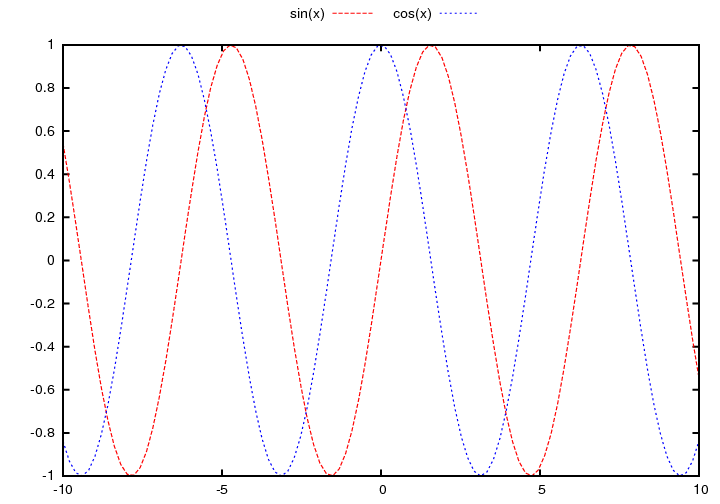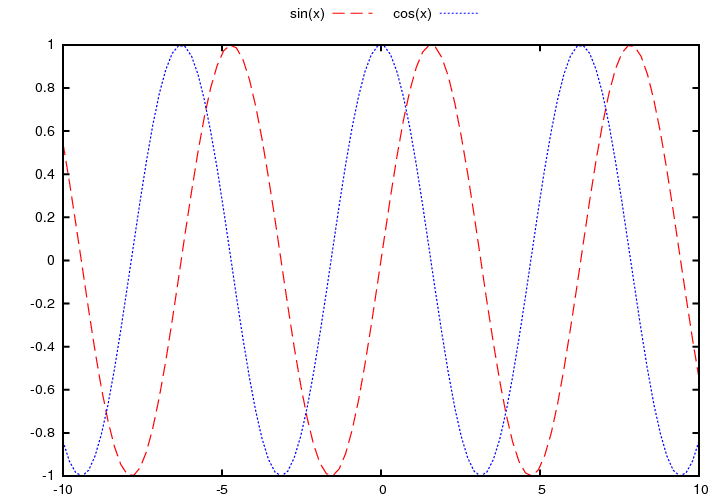pmeerw's blog

28 Apr 2011

## Thu, 28 Apr 2011

### pandaboard

Just got my pandaboard, a dual-core ARM Cortex A9 development board with WLAN, LAN, bluetooth, DVI/HDMI and OpenGL/ES.

posted at: 18:03 | path: /projects/PandaBoard | permanent link

### Implementing Convolutional Codes

A good online tutorial is Charan Langton's Coding and decoding with Convolutional Codes. (Fig. 10 seems incorrect, however, as three arrows go into one state.)

To visualize a Trellis on paper, I found the following useful:Available as: .pdf and .svg

Some code (`test.cpp`) using the it++ library for C++ which illustrates the decoding example given in the tutotial:

```// compile with
//   g++ -Wall -o test test.cpp -litpp
// run with
//   ./test

#include <cstdio>
#include <cassert>

#include <itpp/itbase.h>
#include <itpp/itcomm.h>

// example program, following http://www.complextoreal.com/convo.htm

int main() {
unsigned int invR = 2; // inverse rate
unsigned int m = 4; // memory

itpp::bvec u = "1 0 1 1 "; // input bits

std::cout << "uncoded: " << u << "\n";

itpp::Convolutional_Code cc;
itpp::BPSK bpsk;

itpp::ivec generators;
generators.set_size(invR, false);
generators(0) = 15; // polynomials
generators(1) = 13;

printf("constraint length %u\n", m-1);

cc.set_generator_polynomials(generators, m);
itpp::bvec coded_bits = cc.encode_tail(u);
std::cout << "coded: " << coded_bits << "\n";

itpp::vec symbols = bpsk.modulate_bits(coded_bits);
printf("symbols %d\n", symbols.size());

// channel here
itpp::vec received_symbols = symbols + 0.3*itpp::randn(symbols.size());

std::cout << "decoded: " << decoded_bits << "\n";
}
```

### Change Gnuplot's line type style

I find Gnuplot's default line type styles (solid, dashed, dotted) quite hard to distinguish on small figures:On the left Gnuplot's default line type 2 and 3, on the right the improved version (much better :-))!

My solution is to patch the Postscript code produced by Gnuplot; first the original Postscript code:

```/LT1 {PL [4 dl1 2 dl2] LC1 DL} def
/LT2 {PL [2 dl1 3 dl2] LC2 DL} def
```
and here the patched lines:
```/LT1 {PL [12 dl1 6 dl2] LC1 DL} def
/LT2 {PL [2 dl1 2 dl2] LC2 DL} def
```

Here is the .plot file and the original and patched .eps file.